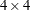## Example 67.4 A Latin Square Design

All of the preceding examples involve designs with completely nested block structures, for which PROC PLAN was especially designed. However, by appropriate coordination of its facilities, a much wider class of designs can be accommodated. A Latin square design is based on experimental units that have a row-and-column block structure. The following example uses the CYCLIC option for a treatment factor tmts to generate a simpleLatin square. Randomizing a Latin square design involves randomly permuting the row, column, and treatment values independently. In order to do this, use the RANDOM option in the OUTPUT statement of PROC PLAN. The example also uses factor-value-settings in the OUTPUT statement. The following statements produce Output 67.4.1:

```title 'Latin Square Design';
proc plan seed=37430;
factors Row=4 ordered Col=4 ordered / noprint;
treatments Tmt=4 cyclic;
output out=LatinSquare
Row cvals=('Day 1' 'Day 2' 'Day 3' 'Day 4') random
Col cvals=('Lab 1' 'Lab 2' 'Lab 3' 'Lab 4') random
Tmt nvals=(      0     100     250     450) random;
quit;
```
```proc sort data=LatinSquare out=LatinSquare;
by Row Col;
proc transpose data= LatinSquare(rename=(Col=_NAME_))
out =tLatinSquare(drop=_NAME_);
by Row;
var Tmt;
proc print data=tLatinSquare noobs;
run;
```

Output 67.4.1 A Randomized Latin Square Design
 Latin Square Design

Row Lab_1 Lab_2 Lab_3 Lab_4
Day 1 0 250 100 450
Day 2 250 450 0 100
Day 3 100 0 450 250
Day 4 450 100 250 0